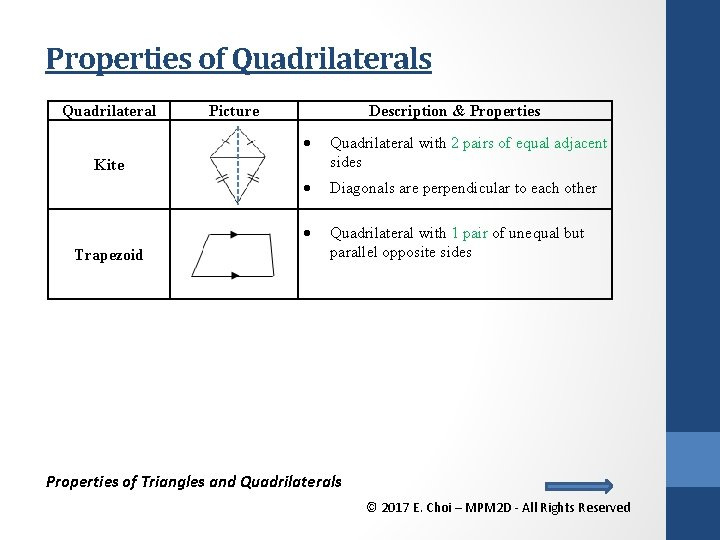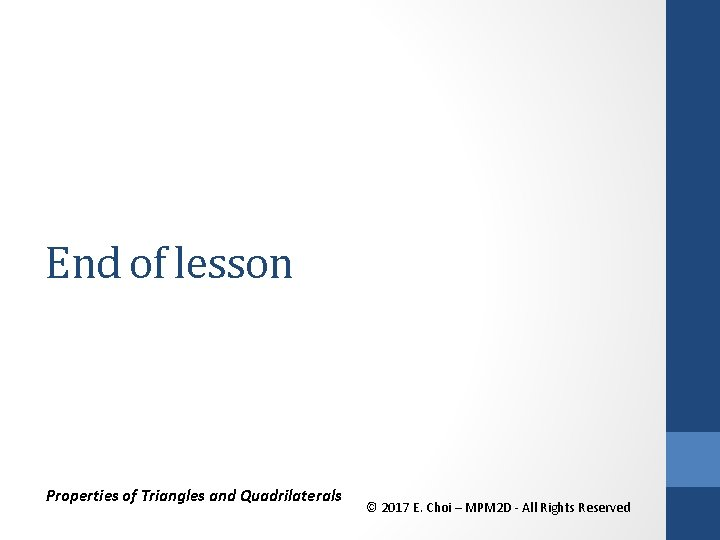• Slides: 24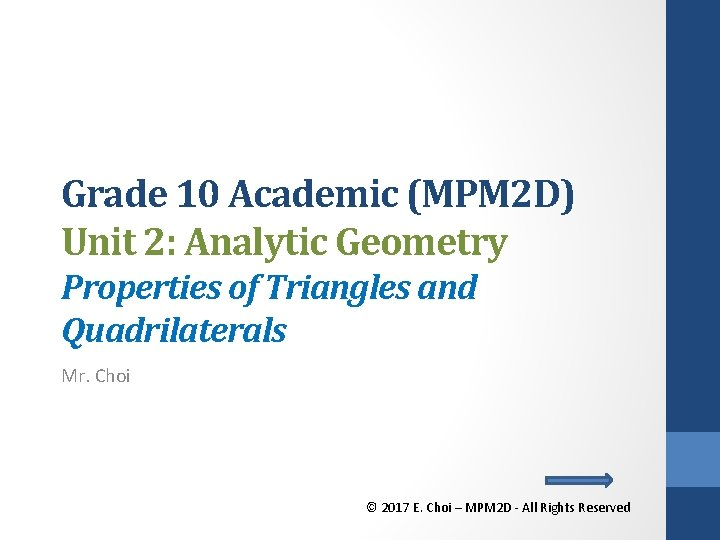Properties of Triangles • Pythagorean Theorem used to find the length of sides of a right triangle Properties of Triangles and Quadrilaterals © 2017 E. Choi – MPM 2 D - All Rights Reserved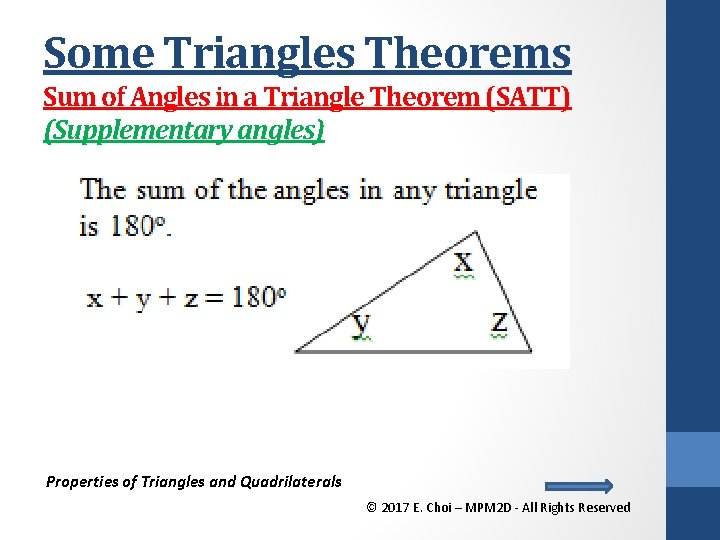Some Triangles Theorems Sum of Angles in a Triangle Theorem (SATT) (Supplementary angles) Properties of Triangles and Quadrilaterals © 2017 E. Choi – MPM 2 D - All Rights Reserved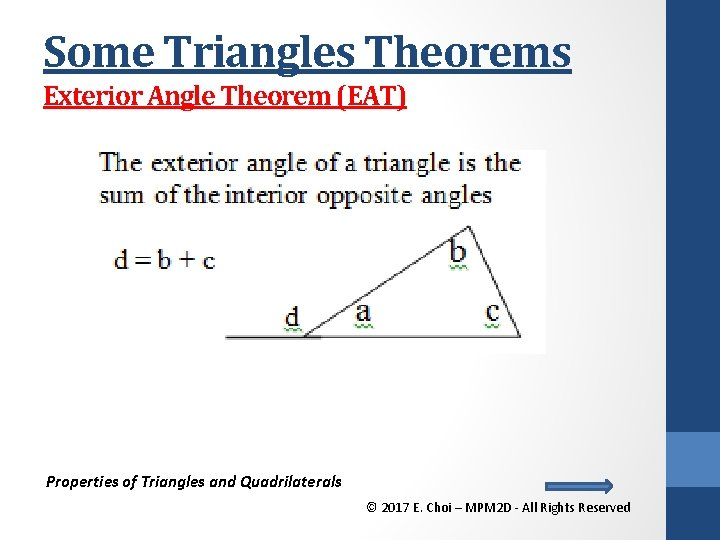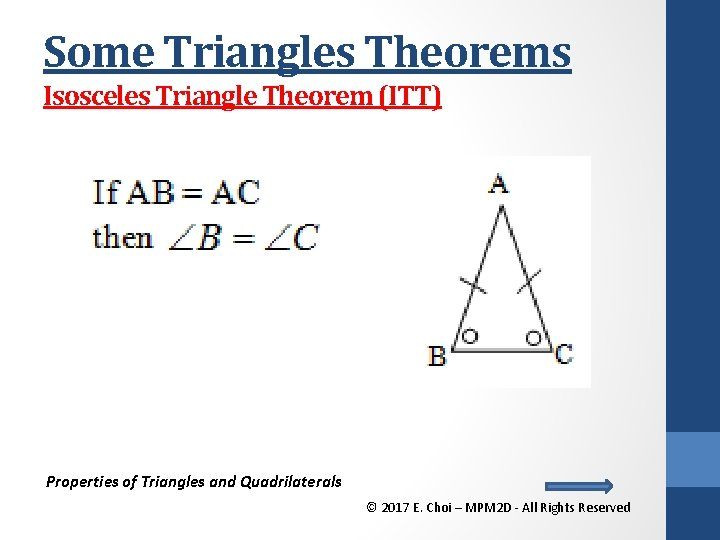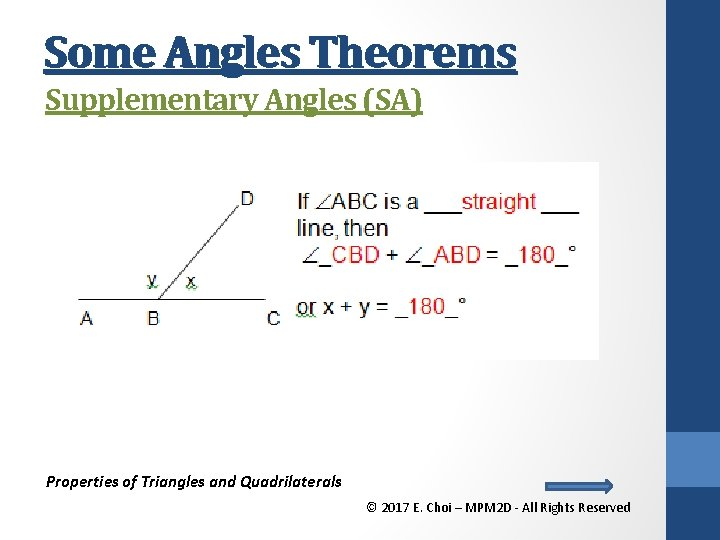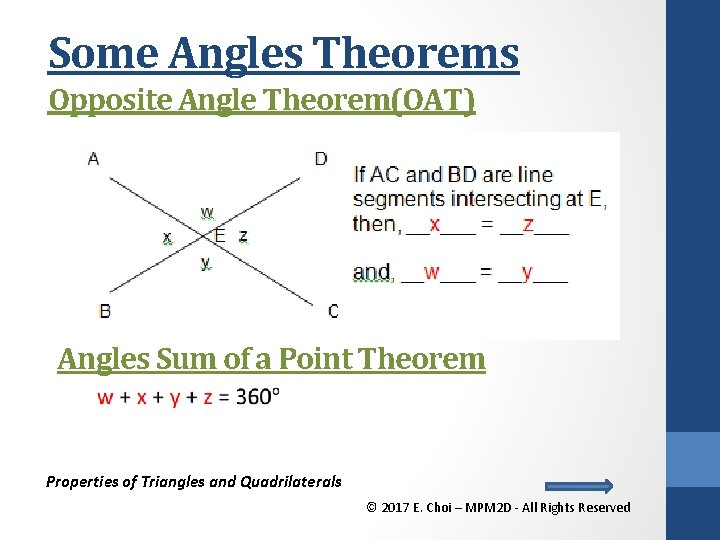Some Angles Theorems Opposite Angle Theorem(OAT) Angles Sum of a Point Theorem Properties of Triangles and Quadrilaterals © 2017 E. Choi – MPM 2 D - All Rights ReservedSome Angles Theorems Sum of Angles in a Triangle Theorem(SATT) (Supplementary angles) Properties of Triangles and Quadrilaterals © 2017 E. Choi – MPM 2 D - All Rights Reserved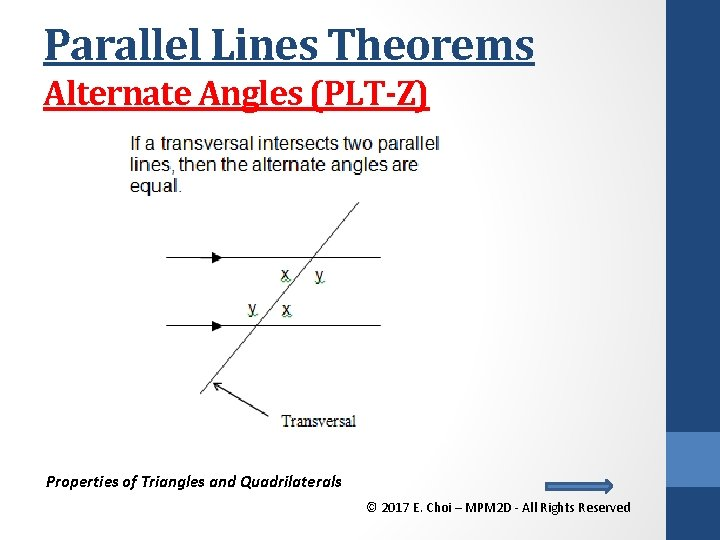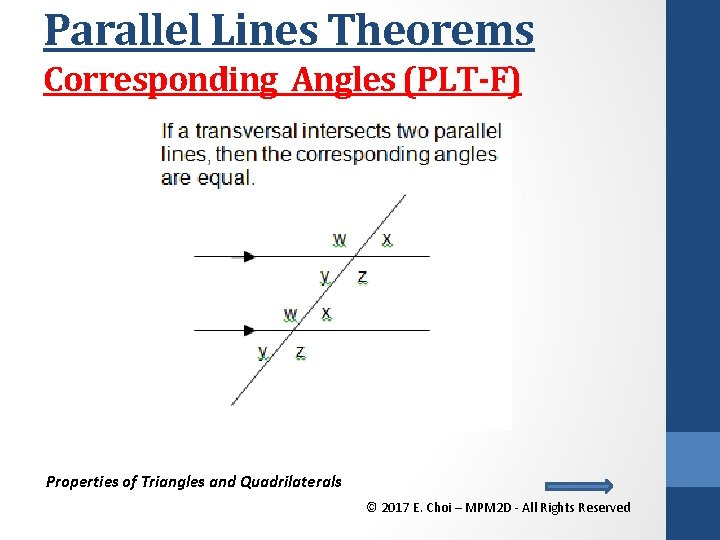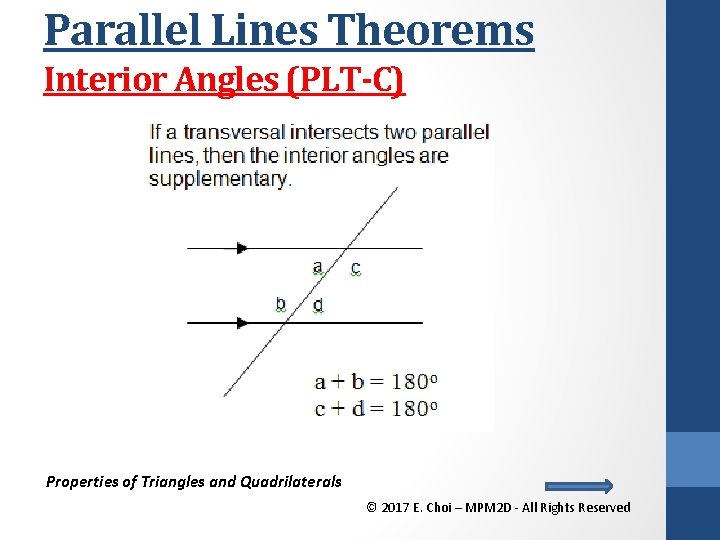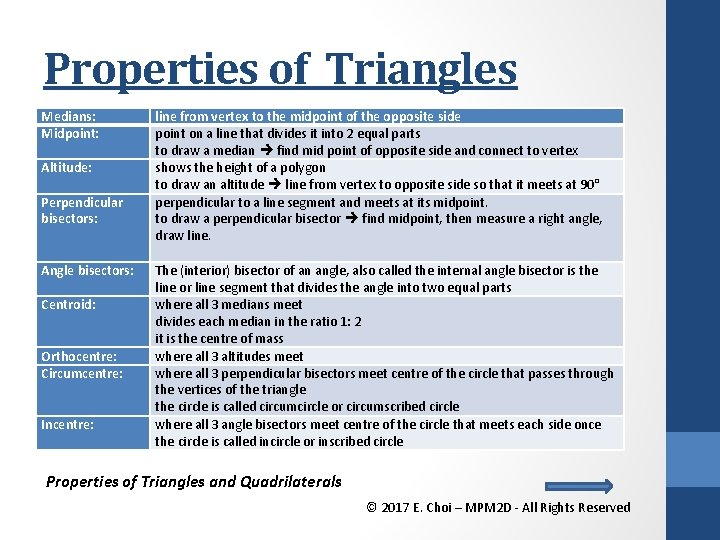Properties of Triangles Medians: Midpoint: Altitude: Perpendicular bisectors: Angle bisectors: Centroid: Orthocentre: Circumcentre: Incentre: line from vertex to the midpoint of the opposite side point on a line that divides it into 2 equal parts to draw a median find mid point of opposite side and connect to vertex shows the height of a polygon to draw an altitude line from vertex to opposite side so that it meets at 90° perpendicular to a line segment and meets at its midpoint. to draw a perpendicular bisector find midpoint, then measure a right angle, draw line. The (interior) bisector of an angle, also called the internal angle bisector is the line or line segment that divides the angle into two equal parts where all 3 medians meet divides each median in the ratio 1: 2 it is the centre of mass where all 3 altitudes meet where all 3 perpendicular bisectors meet centre of the circle that passes through the vertices of the triangle the circle is called circumcircle or circumscribed circle where all 3 angle bisectors meet centre of the circle that meets each side once the circle is called incircle or inscribed circle Properties of Triangles and Quadrilaterals © 2017 E. Choi – MPM 2 D - All Rights ReservedMedian & Centroid Medians: line from vertex to the midpoint of the opposite side Centroid: where all 3 medians meet divides each median in the ratio 1: 2 it is the centre of mass Properties of Triangles and Quadrilaterals © 2017 E. Choi – MPM 2 D - All Rights Reserved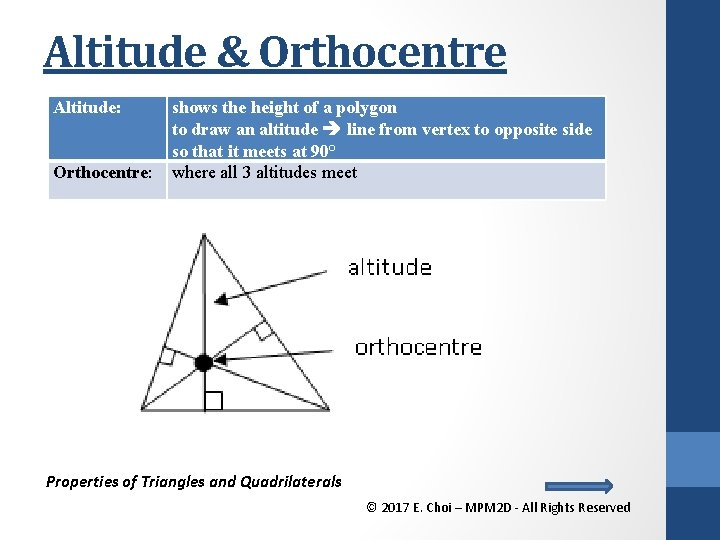Altitude & Orthocentre Altitude: Orthocentre: shows the height of a polygon to draw an altitude line from vertex to opposite side so that it meets at 90° where all 3 altitudes meet Properties of Triangles and Quadrilaterals © 2017 E. Choi – MPM 2 D - All Rights Reserved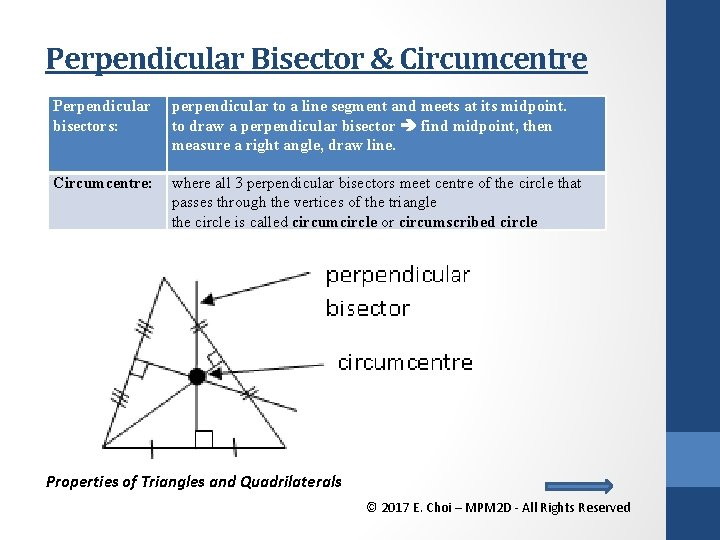Perpendicular Bisector & Circumcentre Perpendicular bisectors: perpendicular to a line segment and meets at its midpoint. to draw a perpendicular bisector find midpoint, then measure a right angle, draw line. Circumcentre: where all 3 perpendicular bisectors meet centre of the circle that passes through the vertices of the triangle the circle is called circumcircle or circumscribed circle Properties of Triangles and Quadrilaterals © 2017 E. Choi – MPM 2 D - All Rights Reserved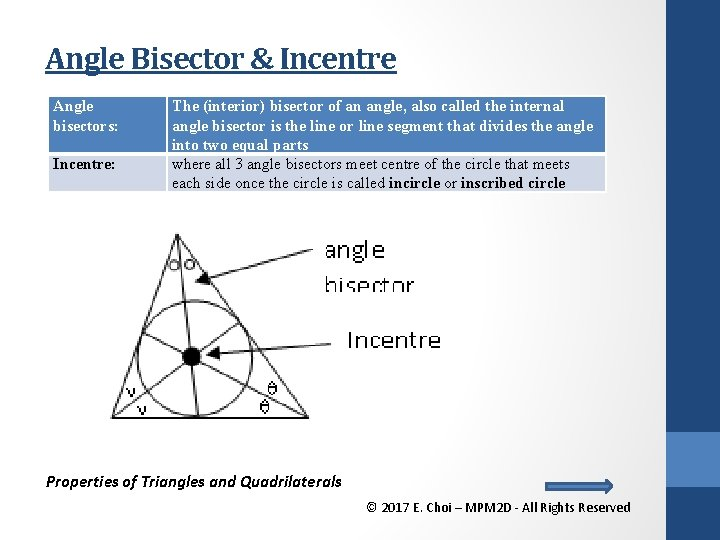Angle Bisector & Incentre Angle bisectors: Incentre: The (interior) bisector of an angle, also called the internal angle bisector is the line or line segment that divides the angle into two equal parts where all 3 angle bisectors meet centre of the circle that meets each side once the circle is called incircle or inscribed circle Properties of Triangles and Quadrilaterals © 2017 E. Choi – MPM 2 D - All Rights Reserved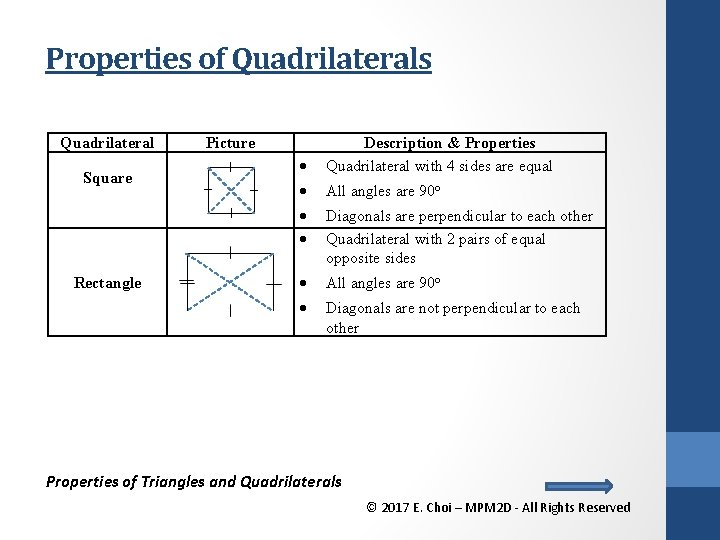Properties of Quadrilaterals Quadrilateral Square Rectangle Picture Description & Properties Quadrilateral with 4 sides are equal All angles are 90 o Diagonals are perpendicular to each other Quadrilateral with 2 pairs of equal opposite sides All angles are 90 o Diagonals are not perpendicular to each other Properties of Triangles and Quadrilaterals © 2017 E. Choi – MPM 2 D - All Rights ReservedProperties of Quadrilaterals Quadrilateral Rhombus Parallelogram Picture Description & Properties Quadrilateral with 4 sides are equal None of angles is 90 o A pair of acute angles and a pair of obtuse angles Diagonals are perpendicular to each other Quadrilateral with 2 pairs of equal opposite sides None of angles is 90 o A pair of acute angles and a pair of obtuse angles Diagonals are not perpendicular to each other Properties of Triangles and Quadrilaterals © 2017 E. Choi – MPM 2 D - All Rights Reserved